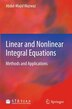# Linear and Nonlinear Integral Equations: Methods and Applications

## byAbdul-Majid Wazwaz

### Pricing and Purchase Info

\$258.95

Earn 1,295 plum® points

Prices and offers may vary in store

HURRY, ONLY 3 LEFT!
Quantity:

In stock online

Ships free on orders over \$25

Not available in stores

### about

Linear and Nonlinear Integral Equations: Methods and Applications is a self-contained book divided into two parts. Part I offers a comprehensive and systematic treatment of linear integral equations of the first and second kinds. The text brings together newly developed methods to reinforce and complement the existing procedures for solving linear integral equations. The Volterra integral and integro-differential equations, the Fredholm integral and integro-differential equations, the Volterra-Fredholm integral equations, singular and weakly singular integral equations, and systems of these equations, are handled in this part by using many different computational schemes. Selected worked-through examples and exercises will guide readers through the text. Part II provides an extensive exposition on the nonlinear integral equations and their varied applications, presenting in an accessible manner a systematic treatment of ill-posed Fredholm problems, bifurcation points, and singular points. Selected applications are also investigated by using the powerful Padé approximants. This book is intended for scholars and researchers in the fields of physics, applied mathematics and engineering. It can also be used as a text for advanced undergraduate and  graduate students in applied mathematics, science and engineering, and related fields. Dr. Abdul-Majid Wazwaz is a Professor of Mathematics at Saint Xavier University in Chicago, Illinois, USA.
Title:Linear and Nonlinear Integral Equations: Methods and ApplicationsFormat:HardcoverDimensions:657 pages, 9.25 × 6.1 × 0 inPublished:September 6, 2011Publisher:Springer Berlin HeidelbergLanguage:English

The following ISBNs are associated with this title:

ISBN - 10:3642214487

ISBN - 13:9783642214486

Look for similar items by category:

BooksScience and NatureMathematics

## Reviews

Table of Contents

PART I: Linear Integral Equations. Preliminaries.- Introductory Concepts of Integral Equations.- Volterra Integral Equations.- Fredholm Integral Equations.- Volterra Integro-Differential Equations.- Fredholm Integro-Differential Equations.- Abel's Integral Equation and Singular Integral Equations.- Volterra-Fredholm Integral Equations.- Volterra-Fredholm Integro-Differential Equations.- Systems of Volterra Integral Equations.- Systems of Fredholm Integral Equations.-Systems of Singular Integral Equations. PART II: Nonlinear Integral Equations. Nonlinear Volterra Integral Equations.- Nonlinear Volterra Integro-Differential Equations.- Nonlinear Fredholm Integral Equations.- Nonlinear Fredholm Integro-Differential Equations.- Nonlinear Singular Integral Equations.- Applications of Integral Equations.

Editorial Reviews

From the reviews:"This is a useful book that concerns solution methods for integral equations of Volterra and Fredholm type of the 1st and 2nd kinds. The book focuses on methods and applications and this sets it apart from other works which have more focus on theory and analysis. The book is therefore accessible to a wide range of researchers and advanced students who will find it answers many of their practical problems of how to get started with a particular approach." (Neville Ford, Zentralblatt MATH, Vol. 1227, 2012)